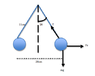# Electrostatic repulsion problem

• jessicak
In summary, when two balls of mass 1.5x10-4kg each carry equal charges and are suspended by identical threads of length 11cm anchored 28cm apart, the charge on each ball can be calculated using the equation q=(mgtan20/k)1/2(0.28m), which results in 6.8x10-8C. However, it is important to carefully read and interpret the problem, as the "anchored" distance may refer to the point of suspension of the threads, rather than the distance between the balls. In this case, the distance between the balls would be 7.5 cm.

## Homework Statement

Suppose that two balls of mass 1.5x10-4kg each carry equal charges and are suspended by identical threads of length 11cm anchored 28cm apart. If each thread makes an angle of 20 degrees with the vertical, what is the charge on each ball?

F=kq2/r2

## The Attempt at a Solution

From a free body diagram of one of the masses:
mg=Tcos20
Fe=Tsin20

which can be combined to Fe = mgtan20

using the above equation:

q=(mgtan20/k)1/2(0.28m)

using this, I get 6.8x10-8C

can some one point out where I'm going wrong?

It is always useful to read the problem carefully and sketch the set-up. Are you sure that those balls are 28 cm apart? What does "anchored" mean? And what are anchored 28 cm apart?

ehild

The attached thumbnail would be my interpretation of the problem, where the masses are "anchored" by repulsion, giving the distance between them to be 28cm. Therefore, by saying anchored, we know that the net force is 0. Am I approaching this the wrong way?

#### Attachments

•Picture 2.png
4.8 KB · Views: 378
English is not my first language, but the dictionary says "Anchor: Fix firmly". The balls are not fixed at definite places, but kept there by the balance of forces.

"two balls of mass 1.5x10-4kg each carry equal charges and are suspended by identical threads of length 11cm anchored 28cm apart"
Does anchor refer to the masses or to the threads?

Anyway, try the other set-up and see if you get the demanded result.

ehild

That would be the problem, thank you.

I forgot to point out that the distance between the masses in your figure can not be 28 cm. (It is 2*11*sin(20°)= 7.5 cm..

ehild

that distance 28 cm is actually b/w the pt. of suspension of the 2 threads...